# Number Mazes

Number mazes are a great way to help children with their counting, and also to practice their problem-solving skills. The puzzles can be solved by following the path from the start of the maze to the end starting from number 0 to 9.

Showing 1-10 of 10 records

•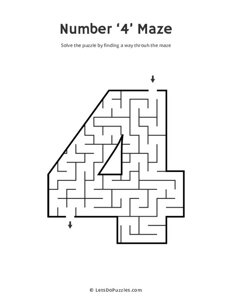Number 4 Maze

Solve the puzzle by finding a way through the Number 4 (four) shaped maze. Download, print and play!

count, math, mazes, number

•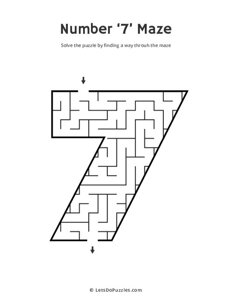Number 7 Maze

Solve the puzzle by finding a way through the Number 7 (seven) shaped maze. Download, print and play!

count, math, mazes, number

•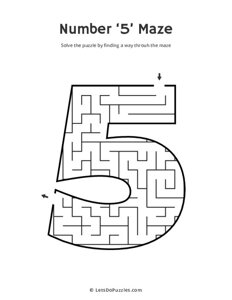Number 5 Maze

Solve the puzzle by finding a way through the Number 5 (five) shaped maze. Download, print and play!

count, math, mazes, number

•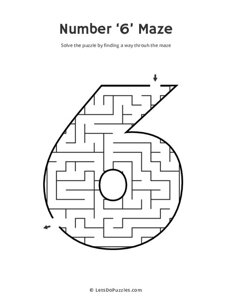Number 6 Maze

Solve the puzzle by finding a way through the Number 6 (six) shaped maze. Download, print and play!

count, math, mazes, number

•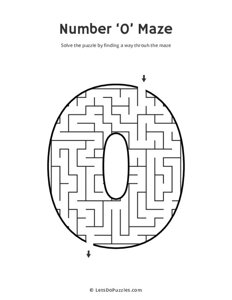Number 0 Maze

Solve the puzzle by finding a way through the Number 0 (zero) shaped maze. Download, print and play!

count, math, mazes, number

•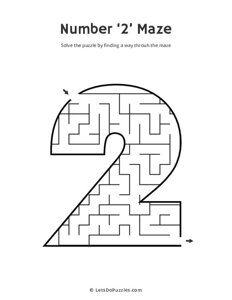Number 2 Maze

Solve the puzzle by finding a way through the Number 2 (two) shaped maze. Download, print and play!

count, math, mazes, number

•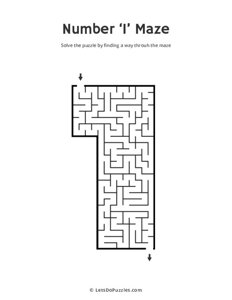Number 1 Maze

Solve the puzzle by finding a way through the Number 1 (one) shaped maze. Download, print and play!

count, math, mazes, number

•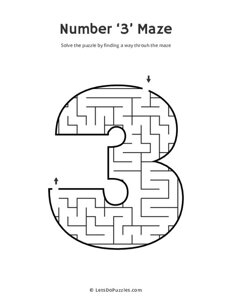Number 3 Maze

Solve the puzzle by finding a way through the Number 3 (three) shaped maze. Download, print and play!

count, math, mazes, number

•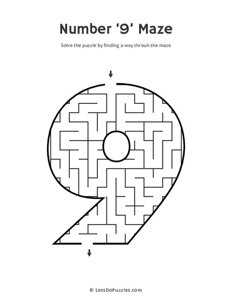Number 9 Maze

Solve the puzzle by finding a way through the Number 9 (nine) shaped maze. Download, print and play!

count, math, mazes, number

•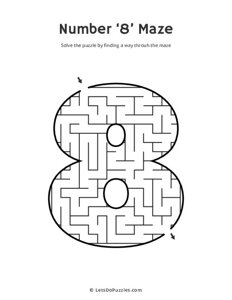Number 8 Maze

Solve the puzzle by finding a way through the Number 8 (eight) shaped maze. Download, print and play!

count, math, mazes, number

## What are the benefits of number mazes?

Number mazes can help children with a number of skills, including:

• Counting: The mazes will help children to count and learn the sequence of numbers.
• Problem solving: The mazes are a fun way for children to practice their problem-solving skills.
• Visual tracking: Children will need to visually track the path through the maze, which can help to develop their visual tracking skills.
• Fine motor skills: Manipulating the pencil to trace the path through the maze can help to develop fine motor skills.

## How to use number mazes?

There are a few different ways that you can use these number mazes:

• Print out the mazes and have your child solve them with a pencil.
• Use the mazes as a worksheet, where your child solves a maze within set time frame.
• Laminate the mazes and use them with a dry erase marker, so that they can be solved over and over again.
• Put the mazes up on a wall or refrigerator and see who can solve them the quickest.

Whichever way you wish to use these number mazes, children are sure to have fun while working on these fun puzzles.